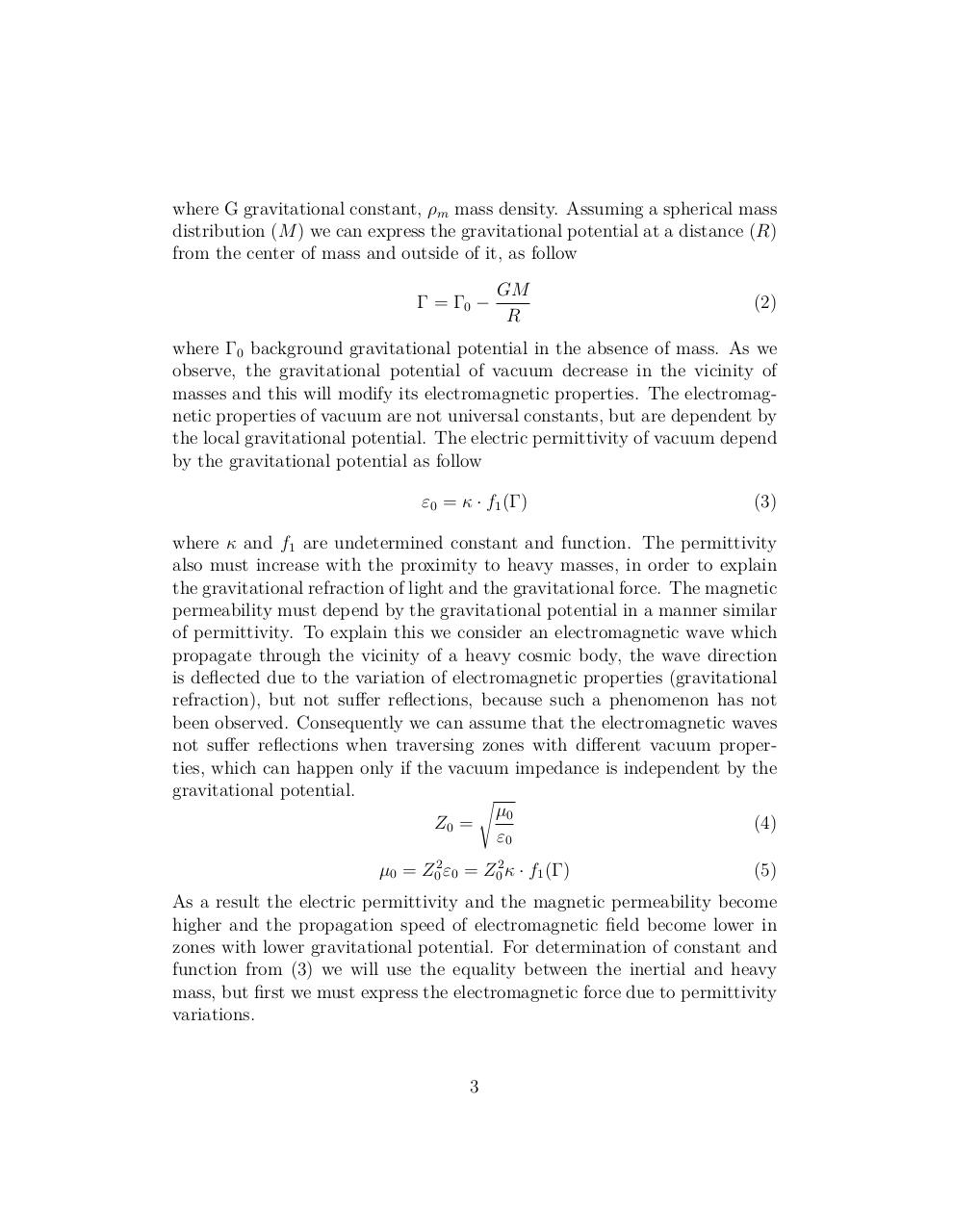# gvac art.pdfPage 1 2 34525

#### Text preview

where G gravitational constant, ρm mass density. Assuming a spherical mass
distribution (M ) we can express the gravitational potential at a distance (R)
from the center of mass and outside of it, as follow
Γ = Γ0 −

GM
R

(2)

where Γ0 background gravitational potential in the absence of mass. As we
observe, the gravitational potential of vacuum decrease in the vicinity of
masses and this will modify its electromagnetic properties. The electromagnetic properties of vacuum are not universal constants, but are dependent by
the local gravitational potential. The electric permittivity of vacuum depend
by the gravitational potential as follow
ε0 = κ · f1 (Γ)

(3)

where κ and f1 are undetermined constant and function. The permittivity
also must increase with the proximity to heavy masses, in order to explain
the gravitational refraction of light and the gravitational force. The magnetic
permeability must depend by the gravitational potential in a manner similar
of permittivity. To explain this we consider an electromagnetic wave which
propagate through the vicinity of a heavy cosmic body, the wave direction
is deflected due to the variation of electromagnetic properties (gravitational
refraction), but not suffer reflections, because such a phenomenon has not
been observed. Consequently we can assume that the electromagnetic waves
not suffer reflections when traversing zones with different vacuum properties, which can happen only if the vacuum impedance is independent by the
gravitational potential.
s
µ0
(4)
Z0 =
ε0
µ0 = Z02 ε0 = Z02 κ · f1 (Γ)

(5)

As a result the electric permittivity and the magnetic permeability become
higher and the propagation speed of electromagnetic field become lower in
zones with lower gravitational potential. For determination of constant and
function from (3) we will use the equality between the inertial and heavy
mass, but first we must express the electromagnetic force due to permittivity
variations.

3